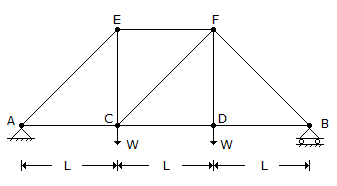# Civil Engineering - UPSC Civil Service Exam Questions

16.

In the truss shown in the given figure which one of the following members has no force induced in it ?A. CD B. CE C. CF D. DF

Explanation:

No answer description available for this question. Let us discuss.

17.

Consider the following statements :
A : An isotropic material is always homogeneous.
R : An isotropic material is one in which all the properties are the same in all the directions
at every point.
Of these statements :

 A. Both A and R are true and R is the correct explanation of A B. Both A and R are true but R is not a correct explanation of A C. A is true but R is false D. A is false but R is true

Explanation:

No answer description available for this question. Let us discuss.

18.

The ratio of pressures between two points A and B located respectively at depths 0.5 m, and 2 m below a constant level of water in a tank is

 A. 1 : 2 B. 1 : 2 C. 1 : 4 D. 1 : 16

Explanation:

No answer description available for this question. Let us discuss.

19.

The acceleration of a particle starting from rest varies with time according to the relation a = - sω2 sin ωt
What is the displacement of this particle at a time t ?

 A. s sin ωt B. sω cos ωt C. sω sin ωt D. - 1/2 (sω2 sin ωt)t2

Explanation:

No answer description available for this question. Let us discuss.

20.

For which one of the following purposes is the double mass curve used ?

 A. Checking on the consistency of precipitation records B. Prediction of annual precipitation C. Defining which periods of storm should be analyzed to obtain the maximum useful information from storm rainfall records D. For estimating the capacity of a reservoir.